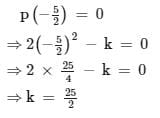Test: Factorisation Factor Theorem

# Test: Factorisation Factor Theorem

Test Description

## 20 Questions MCQ Test Mathematics (Maths) Class 9 | Test: Factorisation Factor Theorem

Test: Factorisation Factor Theorem for Class 9 2023 is part of Mathematics (Maths) Class 9 preparation. The Test: Factorisation Factor Theorem questions and answers have been prepared according to the Class 9 exam syllabus.The Test: Factorisation Factor Theorem MCQs are made for Class 9 2023 Exam. Find important definitions, questions, notes, meanings, examples, exercises, MCQs and online tests for Test: Factorisation Factor Theorem below.
Solutions of Test: Factorisation Factor Theorem questions in English are available as part of our Mathematics (Maths) Class 9 for Class 9 & Test: Factorisation Factor Theorem solutions in Hindi for Mathematics (Maths) Class 9 course. Download more important topics, notes, lectures and mock test series for Class 9 Exam by signing up for free. Attempt Test: Factorisation Factor Theorem | 20 questions in 20 minutes | Mock test for Class 9 preparation | Free important questions MCQ to study Mathematics (Maths) Class 9 for Class 9 Exam | Download free PDF with solutions
 1 Crore+ students have signed up on EduRev. Have you?
Test: Factorisation Factor Theorem - Question 1

### x-a is a factor of p(x) = ax2+bx+c. Which of the following is true?​

Detailed Solution for Test: Factorisation Factor Theorem - Question 1

See if g(x) = x- a
Then g(x) is a factor of p(x)
The zero of polynomial = a
Therefore p(a)= 0

Test: Factorisation Factor Theorem - Question 2

### If x+1 is a factor of x3+3x2+3x+a, then a = ?

Detailed Solution for Test: Factorisation Factor Theorem - Question 2

Given, x3 + 3x2 + 3x + a = 0

and its factor is x + 1 = 0

⇒ x = -1

Put the value in equation i.e. x3 + 3x2 + 3x + a = 0

it becomes = (-1) + 3 (1) + 3(-1) + a = 0

⇒ -1 + 3 - 3 + a = 0

⇒ a = 1

Test: Factorisation Factor Theorem - Question 3

### If (x-2) is the factor of x2 + 2x+ a, find the value of ‘a’.

Detailed Solution for Test: Factorisation Factor Theorem - Question 3

If x-2 is a factor then 2 is the zero of the polynomial
by substituting 2 in the given polynomial,we get
2^2+2(2)+a=0
8+a=0
a=-8

Test: Factorisation Factor Theorem - Question 4

If a polynomial p(x) is divided by a linear divisor (x-a), then the remainder is

Test: Factorisation Factor Theorem - Question 5

The value of p for which x + p is a factor of x2 + px + 3 – p is:

Test: Factorisation Factor Theorem - Question 6

For two polynomials p(x) and q(x), x-a and x-b are their factors, respectively.Which of the following is true?​

Test: Factorisation Factor Theorem - Question 7

Find the value of a such that (x – 2) is the factor of the polynomial x4 + ax3 + 2x2– 3x

Detailed Solution for Test: Factorisation Factor Theorem - Question 7

Zero of the polynomial is x -2 =0 =>x = 2 put the value of x = 2 (2²)² + a(2)³ + 2(2)² - 3(2) = 16 + 8a + 8 - 6 = 18 + 8a = 0 = 8a = -18 = a = -18/8 = a = -9/4

Test: Factorisation Factor Theorem - Question 8

One of the linear factors of ​3x2 + 8x + 5 is

Test: Factorisation Factor Theorem - Question 9

What is the value of b such that x+3 is a factor of x2 - bx -12?

Test: Factorisation Factor Theorem - Question 10

Find the value of ‘a’ so that (x-1) is the factor of x2+a+1

Test: Factorisation Factor Theorem - Question 11

For a polynomial p(x), x-2 is a factor, so p(2) is _______​

Test: Factorisation Factor Theorem - Question 12

x+1 is a factor of p(x) = x3+3x2+3x+1, so what is the value of p(-1)

Test: Factorisation Factor Theorem - Question 13

If (x-1) is a factor of ax – a, then find the value of a​

Detailed Solution for Test: Factorisation Factor Theorem - Question 13

Solution :- f(x) = ax - a……………………..(1)

x - 1 = 0

x = 1

Putting in eq(1)

(1)x - 1 => x - 1

For eg :- if we put x = 100

Putting in eq(1)

(100)x - 100 => x - 100

So, all the values of a

Test: Factorisation Factor Theorem - Question 14

For a polynomial p(x), p(-1) and p(2) are both equal to zero .So, we can conclude that,

Test: Factorisation Factor Theorem - Question 15

For a polynomial, x2 – 3x +2, which of the following is a factor ?

Test: Factorisation Factor Theorem - Question 16

If (2x + 5) is a factor of 2x2 – k, then the value of k is.

Detailed Solution for Test: Factorisation Factor Theorem - Question 16

Let p(x) = 2x2 − k
Since, (2x+5) is a factor of p(x), then by factor
theorem,Test: Factorisation Factor Theorem - Question 17

(x + 2) is a factor of 2x3 + 5x2 - x - k. The value of k is:

Test: Factorisation Factor Theorem - Question 18

If x-2 is a factor of ax2-x-6, so what should be the value of a?

Test: Factorisation Factor Theorem - Question 19

Find the value of p(2) if p(x) has (x-2) as its factor

Test: Factorisation Factor Theorem - Question 20

Which of the following polynomials has -3 as a zero?

## Mathematics (Maths) Class 9

88 videos|397 docs|109 tests
 Use Code STAYHOME200 and get INR 200 additional OFF Use Coupon Code
Information about Test: Factorisation Factor Theorem Page
In this test you can find the Exam questions for Test: Factorisation Factor Theorem solved & explained in the simplest way possible. Besides giving Questions and answers for Test: Factorisation Factor Theorem, EduRev gives you an ample number of Online tests for practice

## Mathematics (Maths) Class 9

88 videos|397 docs|109 tests